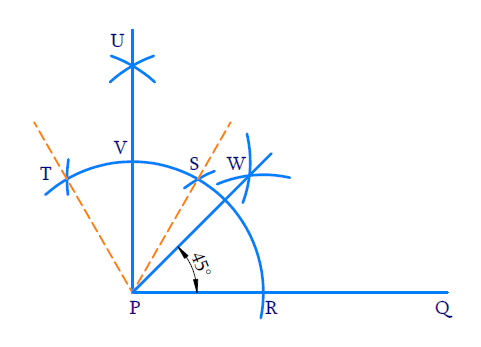# Ex.11.1 Q2 Constructions Solution - NCERT Maths Class 9

Go back to  'Ex.11.1'

## Question

Construct an angle of $$45^\circ$$ at the initial point of a given ray and justify the construction.

Video Solution
Constructions
Ex 11.1 | Question 2

## Text Solution

Reasoning:

We need to construct two adjacent angles each of $$60^\circ$$ degrees and bisect the second one to construct $$90^\circ$$ . Then bisect the $$90^\circ$$ angle to get $$45^\circ$$

\begin{align}\frac{60^{0}+\frac{60^{0}}{2}}{2}=45^{0}\end{align}

Steps of Construction:(i) Draw ray $$PQ$$.

(ii) To construct an angle of $$60^{o}$$ . With $$P$$ as center draw a wide arc of any radius to intersect the ray at $$R$$. With $$R$$ as center and same radius draw an arc to intersect the initial one at $$S$$. $$\angle {SPR}=60^{\circ}$$ .

(iii) To construct adjacent angle of $$60^{\circ}$$ .

With $$S$$ as centre and same radius draw an arc to intersect the previous arc at $$T$$

$\angle {TPS}=60^{\circ}$

(iv) To bisect $$\angle {TPS}$$

With $$T$$ and $$S$$ as centre and same radius, draw arcs to intersect each other at $$U$$.

\begin{align}\angle {UPS}=\frac{1}{2} \angle {TPS}=30^{\circ}\end{align}

(v) Join $$P$$ and $$U$$ to intersect the initial arc at $$V$$.

\begin{align} \angle {UPQ} &=\angle {UPS}+\angle {SPR} \\ &=30^{\circ}+60^{\circ} \\ &=90^{\circ} \end{align}

(vi) To bisect $$\angle {UPQ}$$

With $$R$$ and $$V$$ as centers and radius greater than half of $$RV$$, draw arc to intersect each other at $$W$$. Join $$PW$$. $$PW$$ is the angle bisector of $$\angle U P Q$$

\begin{align} \angle {WPQ} &=\frac{1}{2} \angle {UPQ} \\ &=\frac{1}{2} \times 90^{\circ} \\ &=45^{\circ} \end{align}

(vii) Ray $$PW$$ forms an angle of $$45^{\circ}$$ with ray $$PQ$$ at the initial point.

Learn from the best math teachers and top your exams

• Live one on one classroom and doubt clearing
• Practice worksheets in and after class for conceptual clarity
• Personalized curriculum to keep up with school